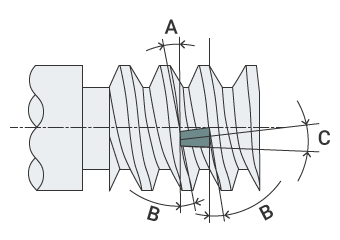# Drilling Formulas

This page introduces formulas for calculating basic parameters necessary for drilling. The figures obtained from the calculation are for reference only. The conditions for machining depend on the machine tool you are using. Use the optimal conditions according to your actual machining circumstances.

## Cutting Speed (vc)

• π (3.14): Circular constant
• DC (mm): Drill diameter
• n (min-1): Spindle speed
memo

This formula is used to calculate the cutting speed from the drill diameter and the spindle speed.
Example:
Drill diameter (DC) = 10 mm
Spindle speed (n) = 1500 min-1
In this case, the cutting speed (vc) is approximately 47.1 m/min.

## Spindle Feed (vf)

• fr (mm/rev): Feed per revolution (feed rate)
• n (min-1): Spindle speed
memo

This formula is used to calculate the spindle (z-axis) feed from the feed per revolution (feed rate) and the spindle speed.
Example:
Feed per revolution (fr) = 0.3 mm/rev
Spindle speed (n) = 1500 min-1
In this case, the spindle (z-axis) feed (vf) is 450 mm/min.

## Machining Time (Tc)

• ld (mm): Drilling depth
• i: Number of holes
• n (min-1): Spindle speed
• fr (mm/rev): Feed per revolution (feed rate)
memo

This formula is used to calculate the machining time from the drilling depth, the number of holes, the spindle feed, and the feed per revolution.
Example of drilling into SCM430 steel:
Drilling depth (ld) = 35 mm
Number of holes (i) = 1
Spindle speed (n) = 1500 min-1
Feed per revolution (fr) = 0.1 mm/rev
In this case, the machining time (Tc) is approximately 14 seconds (0.233 mm/min).

• P (mm): Pitch
• d (mm): Effective diameter of thread
memoA Lead angle (α°) B Flank angle (β°) C Inclination angle of sheet or holder (θ°)

This formula is used to calculate the lead angle of a screw thread.